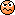Question on Calculating Coulomb force in VECTOR FORM

mhrob24

Homework Statement
Point charges q1 = 14 µC and q2 = −60 µC are fixed at r1 = (5.0î − 4.0ĵ) m and r2 = (9.0î + 7.5ĵ) m.

What is the force (in N) of q2 on q1?
Homework Equations
F = ke(Qq/r^2)
The only thing tripping me up here is that the answer needs to be in vector form. If the question was asking for the scalar form, then I would just find the distance between the charges (plot the charges according to their vector coordinates, then use pythagorean theorem to find the distance between the two. However, I believe (could be wrong here) that in order to get the vector form, I must calculate the force like I normally would for the scalar version, but then I would have to multiply the force by the unit vector representing the distance between the 2 charges. Here is my work done for this problem:However, when I enter that vector at the bottom of my page into webassign (online HW platform), it is incorrect. I know the Force calculation is 100% correct so It has to be something with my unit vector, but I don't see the problem. The x-distance between the two charges is 4m and the y distance between the two charges is 11.5m right? So the vector representing the direct distance between the two charges is < 4.0, 11.5>. Then the unit vector is just this vector divided by its magnitude (sqrt(148.25)). I don't see where I'm going wrong.....

Related Introductory Physics Homework Help News on Phys.org

TSny

Homework Helper
Gold Member
I haven't checked any of the arithmetic. But, without doing any calculation, you should be able to deduce the signs of the x and y components of the force.

•mhrob24

mhrob24

Lmao. You're right. My signs of the components of the force vector were backwards. Q2 will attract Q1 so Q1 will accelerate in both the POSITIVE x and y directions. I had both components of the force vector as negative. I switched the signs and got the correct answer on Webassign. I spent like an hour trying to figure out what was going onThank you!

•TSny

TSny

Homework Helper
Gold Member
Yes. When you express the force vector in terms of a magnitude and a unit vector in the direction of the force, the magnitude part should be a positive number. When finding the magnitude of the force, just use the magnitudes (absolute values) of the charges in Coulomb's law."Question on Calculating Coulomb force in VECTOR FORM"

Physics Forums Values

We Value Quality
• Topics based on mainstream science
• Proper English grammar and spelling
We Value Civility
• Positive and compassionate attitudes
• Patience while debating
We Value Productivity
• Disciplined to remain on-topic
• Recognition of own weaknesses
• Solo and co-op problem solving